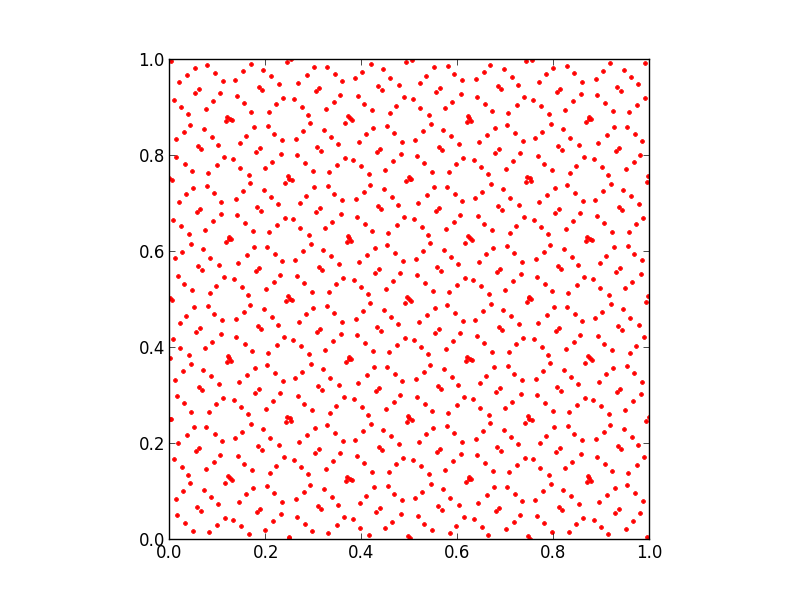53

4

17

11

# The Sobol module for JuliaThis module provides a free Julia-language Sobol low-discrepancy-sequence (LDS) implementation. This generates "quasi-random" sequences of points in N dimensions which are equally distributed over an N-dimensional hypercube.

The advantage of an LDS over truly random (or pseudo-random) numbers is that an LDS (which is not random) tends to be more evenly distributed for finite numbers of points. This is used in quasi-Monte Carlo methods in order to accelerate convergence compared to traditional Monte Carlo methods employing random sequences.

It can be installed using the Julia package manager via `Pkg.add("Sobol")`.

## Algorithm

This is an independent implementation, originally by Steven G. Johnson, of the algorithm for generation of Sobol sequences in up to 21201 dimensions described in:

Originally implemented in C in 2007 as part of the NLopt library for nonlinear optimization, the code was subsequently converted by Ken-B into pure Julia with roughly the same performance.

It is important to emphasize that SGJ's implementation was based on the mathematical description of the algorithms only, and was done without reference to the Fortran code from the Trans. Math. Soft. (TOMS) papers. The reason is that TOMS code is not free/open-source software (it falls under restrictive ACM copyright terms). (SGJ did re-use a table of primitive polynomials and coefficients from the TOMS code, but since this is merely a tabulation of mathematical facts it is not copyrightable.) SGJ's implementation in NLopt, along with this Julia translation, is free/open-source software under the MIT ("expat") license.

Direction numbers used were derived from the file http://web.maths.unsw.edu.au/~fkuo/sobol/new-joe-kuo-6.21201

Technically, we implement a 32-bit Sobol sequence. After 232-1 points, the sequence terminates, and subsequently our implementation returns pseudo-random numbers generated by the Mersenne Twister algorithm. In practical applications, however, this point is rarely reached.

## Usage

To initialize a Sobol sequence `s` in `N` dimensions (`0 < N < 21201`), use the `SobolSeq` constructor:

``````using Sobol
s = SobolSeq(N)
``````

Then

``````x = next!(s)
``````

returns the next point (a `Vector{Float64}`) in the sequence; each point lies in the hypercube [0,1]N. You can also compute the next point in-place with

``````next!(s, x)
``````

where `x` should be a `Vector` of length `N` of some floating-point type (e.g. `Float64`, `Float32`, or `BigFloat`).

You can also use a `SobolSeq` as an iterator in Julia:

``````for x in SobolSeq(N)
...
end
``````

Note, however, that the loop will never terminate unless you explicitly call `break` (or similar) in the loop body at some point of your choosing.

We also provide a different `SobolSeq` constructor to provide an `N`-dimensional Sobol sequence rescaled to an arbitrary hypercube:

``````s = SobolSeq(lb, ub)
``````

where `lb` and `ub` are arrays (or other iterables) of length `N`, giving the lower and upper bounds of the hypercube, respectively. For example, `SobolSeq([-1,0,0],[1,3,2])` generates points in the box [-1,1]×[0,3]×[0,2]. (Although the generated points are `Float64` by default, in general the precision is determined by that of `lb` and `ub`.)

If you know in advance the number `n` of points that you plan to generate, some authors suggest that better uniformity can be attained by first skipping the initial portion of the LDS. In particular, we skip 2ᵐ−1 for the largest `m` where `2ᵐ-1 ≤ n` (see Joe and Kuo, 2003 for a similar suggestion). This facility is provided by:

``````skip(s, n)
``````

Skipping exactly `n` elements is also possible:

``````skip(s, n, exact=true)
``````

`skip` returns `s`, so you can simply do `s = skip(SobolSeq(N))` or similar.

## Example

Here is a simple example, generating 1024 points in two dimensions and plotting them with the PyPlot package. Note the highly uniform, nonrandom distribution of points in the [0,1]×[0,1] unit square!

``````using Sobol
using PyPlot
s = SobolSeq(2)
p = reduce(hcat, next!(s) for i = 1:1024)'
subplot(111, aspect="equal")
plot(p[:,1], p[:,2], "r.")
``````## Author

This module was written by Steven G. Johnson.

01/24/2014

1 day ago

53 commits